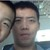# 青岛版数学九年级下册第五章第三节二次函数的定义课件

• 10人评价）
• 98人阅读
• 上传者：王玉冬

### 相关文档推荐2积分 下载
```   你知道吗？

函数

          
一次函数       反比例函数     二次函数
k
y=kx+b (k≠0) y  k    0.
正比例函数           x
y=kx(k≠0)    双曲线
一条直线

你准备好了吗？
有上面的四个问题所列出的函数解析式
分别是：
（1） y=πx2    (2) y=x2-2x
(3)   y=x2+0.5x+0.06

（4）  y=1200x2+2400x+1200

3
4

≠0               =0 ≠0
=0 ≠0 =0

2
y=  3   x x＞0
4
1.下列函数中,哪些是二次函数？
（否）
(1）y=3(x-1)²+1; （是）       1
(2).y  x  .
x
怎么
(3) s=3-2t². （是）      1
判断                  (4).y   （否）.
x2  x

?   (5)y=(x+3)²-x². （否）(6)v=10πr² （是）
(7) y=  x²+ x³+25 （否）

(8)y=2²+2x  （否）
知识的升华

2

2
(1) k为何值时，y是x的一次函数？
（2）k为何值时，y是x的二次函数？

解（1）根据题意得       k 2  k  0

k  0
当 k=1 ，y是x的一次函数。

（  ） 当   2      时
2     k  k  0
y是x的二次函数。

k  0且k  1

2
y= 1  x +4x
2
1.二次函数的定义
2.列二次函数解析式
A组P25 1，3

B组  P26 1  ，2

再见!```
2积分下载